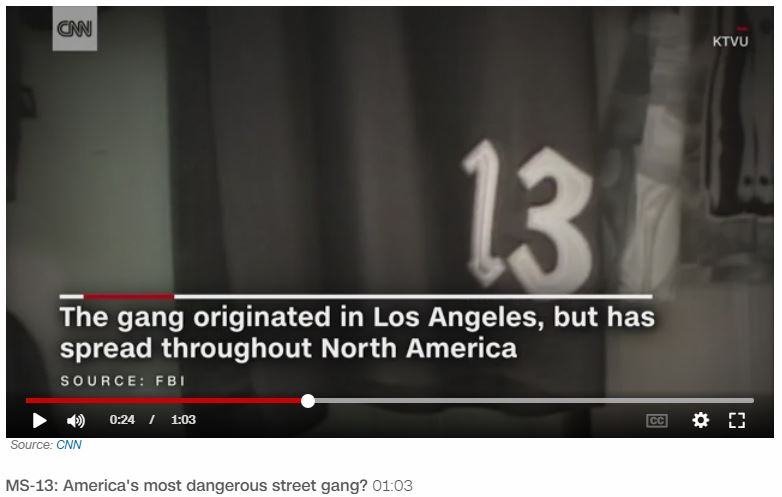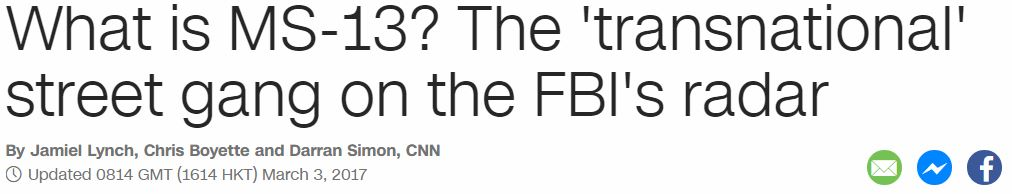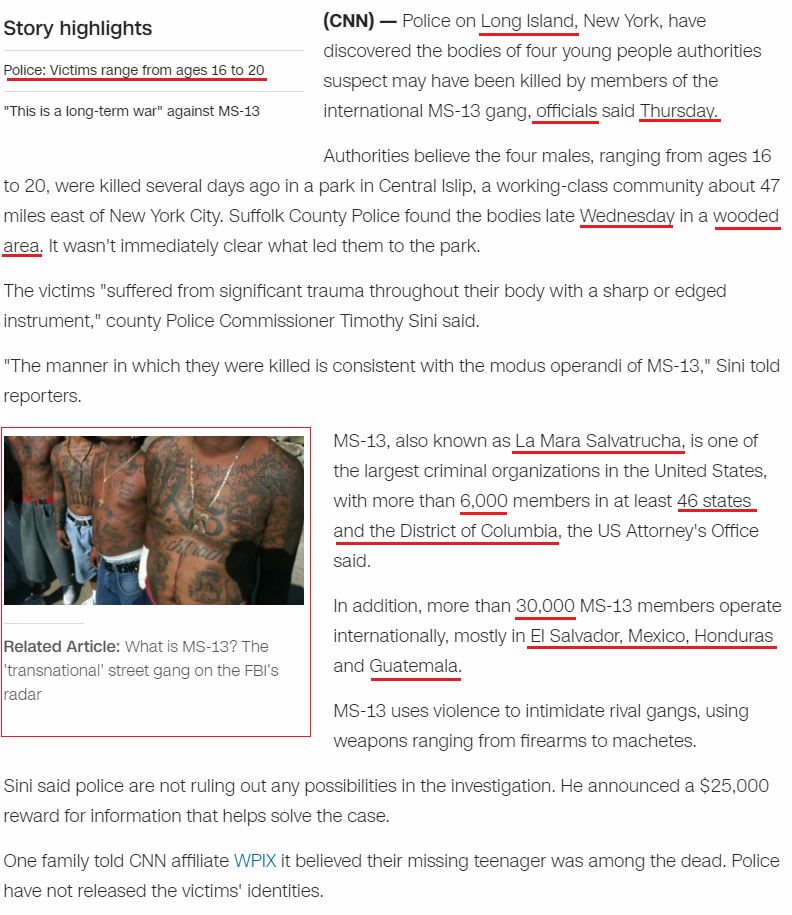## Cipher## Apr 15, 2017

### MS-13, 4 bodies found in New York - 55/46/33

On April 14th CNN released a story that has very interesting connections to the last time they talked about MS-13, this story is mostly centered around 13 and 55 which is also encoded into the date distance between these storiesLength of the video is 1:03.. which is "Sixty three seconds" = 8+18+3+7+2 + 7+19+9+22+22 + 8+22+24+12+13+23+8 = 227 (Reverse Ordinal)

This comes later in the article but I want to start with all of the stuff connecting to number 13
The gang, which federal authorities said is a "transnational criminal enterprise," became so powerful that the FBI started a MS-13 National Gang Task Force in 2004.
2017 is 13 years later... initials of this group also add to 13 - MS = 5+8 = 13 (Reverse Reduction)
In this article you will see a related story that came out on March 3rd, measuring from that story we see this coming on the 41st day (13th prime number) - Suffolk County Police found the bodies late Wednesday in a wooded area.
Wooded area = 23+15+15+4+5+4 + 1+18+5+1 = 91 (English Ordinal) - 13th triangular number is 91If we exclude the end date we see that this is a span of 5 weeks and 5 days (40 days)

The victims were found in "Wooded area" which connects to 55 through 46, and you will see plenty of 46 coded in this story as well
They were found on "Wednesday" = 5+5+4+5+5+10+4+1+7 = 46 (Single Reduction)
wooded area = 5+6+6+4+5+4 + 1+9+5+1 = 46 (Full Reduction)
Also 41 connects to 46 as well - forty one = 6+6+9+2+7 + 6+5+5 = 46 (Full Reduction)
forty six = 6+6+9+2+7 + 10+9+6 = 55 (Single Reduction)

In the picture below you see headline from March 3rd - Police = 7+6+3+9+3+5 = 33 (Full Reduction) - the headline from yesterday starts with the word "Police"Here is a screenshot from April 14th articleThis took place on "Long Island" = 6+3+4+2 + 9+8+6+8+4+5 = 55 (Reverse Reduction)
"officials" = 3+3+3+9+6+9+8+6+8 = 55 (Reverse Reduction) came out with the report on "Thursday" = 7+1+6+9+8+5+8+2 = 46 (Reverse Reduction)
Every time you see a word with 46 gematria in this story you have a direct link to 55 as I already mentioned - "forty six" = 6+6+9+2+7 + 10+9+6 = 55 (Single Reduction)
Note that victims range from 16 to "twenty" = 7+4+22+13+7+2 = 55 (Reverse Ordinal)
(Sixteen = 33, connecting to the "Police (33)", the day they were found also had 33 numerology 4/12/17 = 33) , "Thirty three" = 156 (English Ordinal) - ("four bodies" = 156 (Reverse Ordinal), "four bodies found" = 1056 (English Extended)

Maybe I should mention that this story is released on a date with 55 numerology (4+14+20+17 = 55, which is 2 days after the bodies were found, "two days later" = 2+5+6 + 4+1+7+10 + 3+1+2+5+9 = 55 (Single Reduction)
MS-13 is also know as "La Mara Salvatrucha" = 3+1 + 4+1+9+1 + 1+1+3+4+1+2+9+3+3+8+1 = 55 (Full Reduction)
This group originated in "Los Angeles" = 3+6+10 + 1+5+7+5+3+5+10 = 55 (Single Reduction)

They also start throwing a few numbers and states that connect well to all of this
"with more than 6,000 members in at least 46 states and the District of Columbia, the US Attorney's Office said."
"six thousand" = 1+9+6 + 2+8+6+3+1+1+5+4 = 46 (Full Reduction)
"Forty six states and the District of Columbia" = 21+12+9+7+2 + 8+18+3 + 8+7+26+7+22+8 + 26+13+23 + 7+19+22 + 23+18+8+7+9+18+24+7 + 12+21 + 24+12+15+6+14+25+18+26 = 555 (Reverse Ordinal)
"In addition, more than 30,000 MS-13 members operate internationally, mostly in El Salvador, Mexico, Honduras and Guatemala."
"Thirty thousand" = 100+8+9+80+100+400 + 100+8+50+200+90+1+40+4 = 1190 (Jewish)
"Thirty thousand members" = 7+1+9+9+7+2 + 7+1+3+6+8+8+4+5 + 5+4+5+7+4+9+8 = 119 (Reverse Reduction)
These numbers also connect to the date of release - "Fourteenth of April" = 6+60+300+90+200+5+5+50+200+8 + 60+6 + 1+70+90+9+30 = 1190 (English Extended)

"El Salvador" = 5+3 + 10+1+3+4+1+4+6+9 = 46 (Single Reduction)
"Honduras" = 8+6+5+4+3+9+1+10 = 46 (Single Reduction)
"Guatemala" = 7+3+1+2+5+4+1+3+1 = 27 (Full Reduction)"twenty seven" = 2+5+5+5+2+7 + 10+5+4+5+5 = 55 (Single Reduction), "forty six" = 6+6+9+2+7 + 10+9+6 = 55 (Single Reduction)

And we have one 33 standing out for good measure "Mexico" = 4+5+6+9+3+6 = 33 (Full Reduction)

I can probably find more stuff but I think this is enough for Google to take some more followers from my blog ;) It's really funny to me how they love reading my stuff